# Chemical Quantities Yes you will need a calculator

• Slides: 54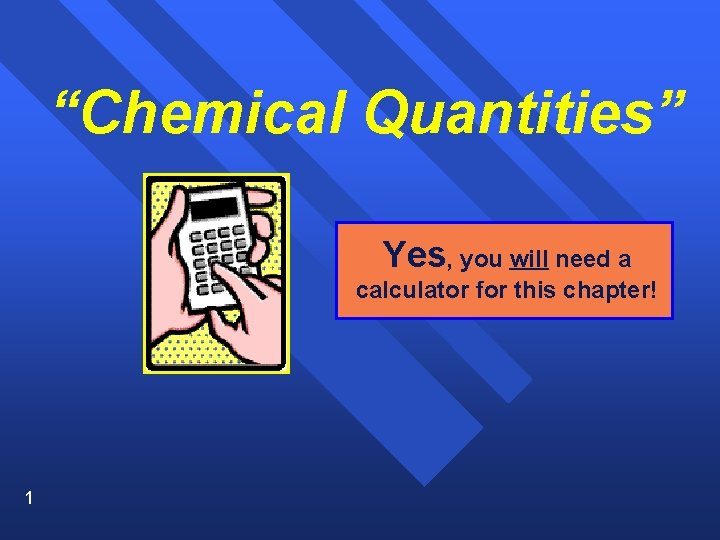“Chemical Quantities” Yes, you will need a calculator for this chapter! 1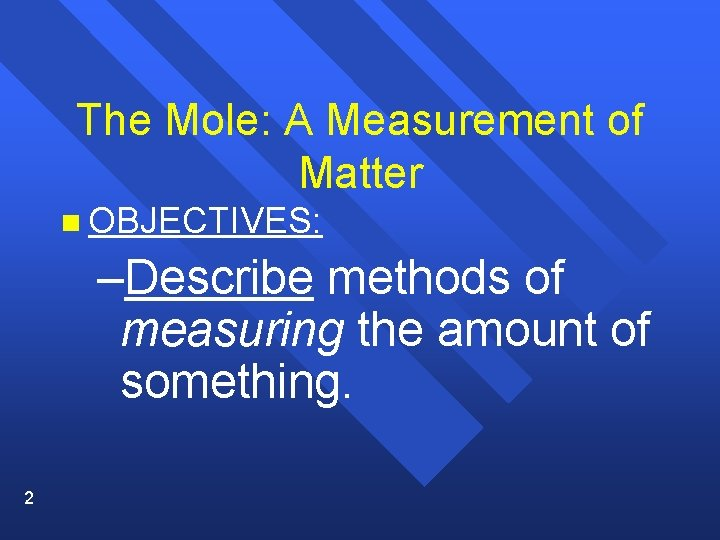The Mole: A Measurement of Matter n OBJECTIVES: –Describe methods of measuring the amount of something. 2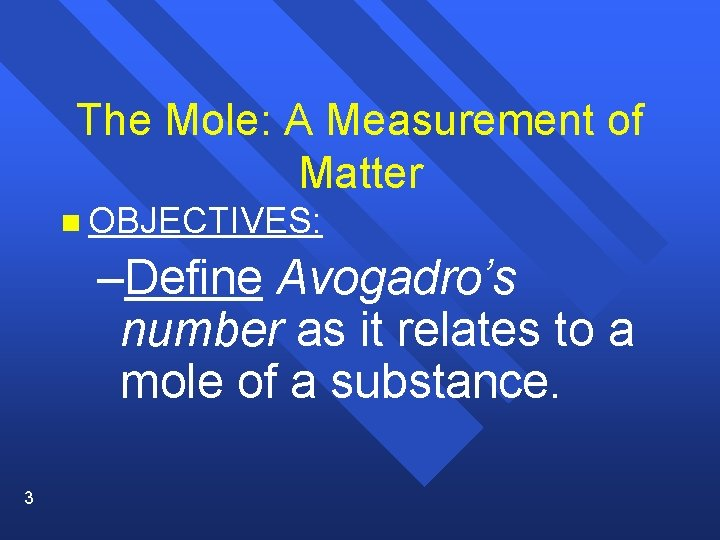The Mole: A Measurement of Matter n OBJECTIVES: –Define Avogadro’s number as it relates to a mole of a substance. 3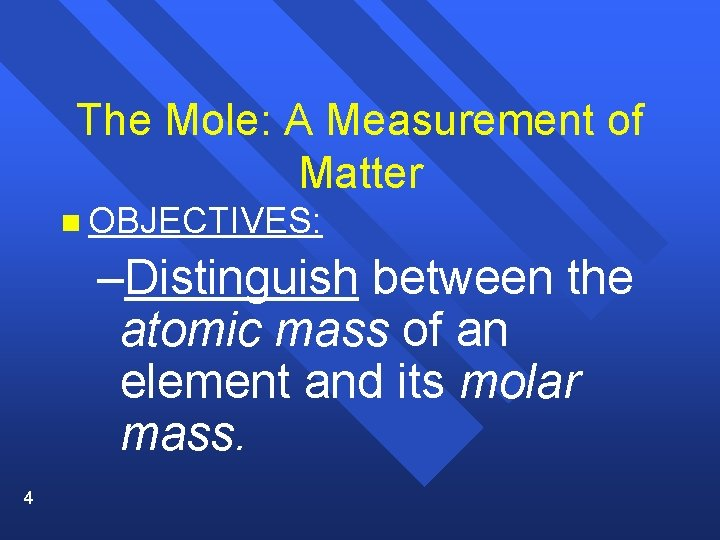The Mole: A Measurement of Matter n OBJECTIVES: –Distinguish between the atomic mass of an element and its molar mass. 4The Mole: A Measurement of Matter n OBJECTIVES: –Describe how the mass of a mole of a compound is calculated. 5“Chemical Quantities” Yes, you will need a calculator for this chapter! 6How do we measure items? You can measure mass, § or volume, § or you can count pieces. § 7 § We measure mass in grams. § We measure volume in liters. § We count pieces in MOLES.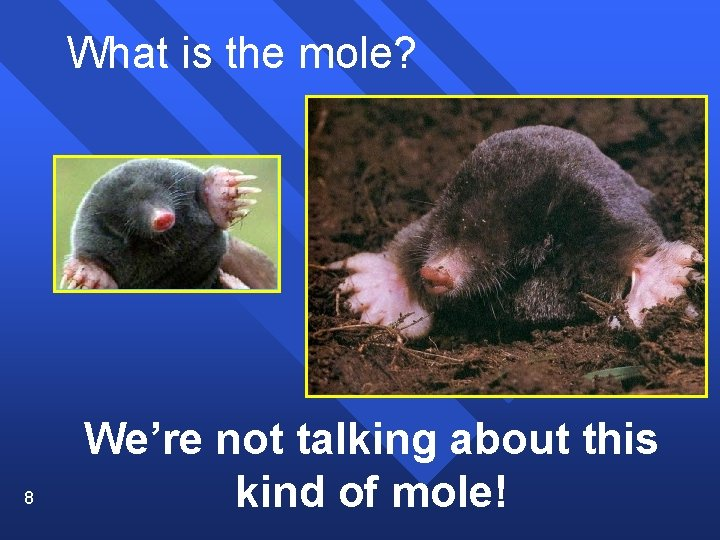What is the mole? 8 We’re not talking about this kind of mole!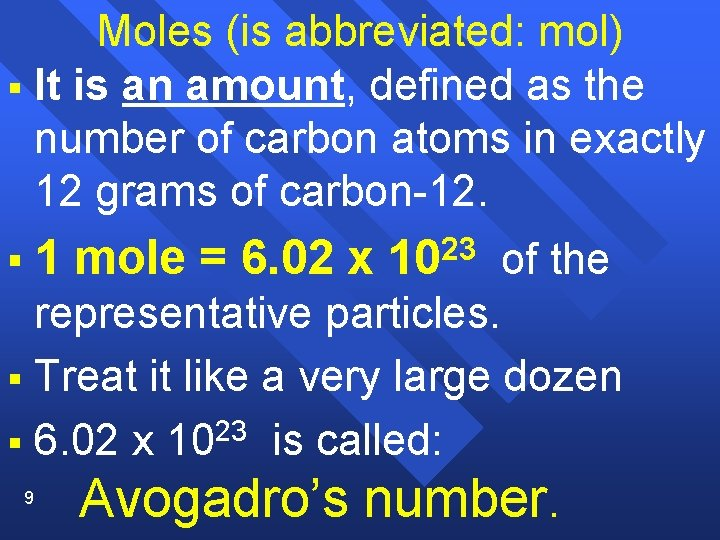Moles (is abbreviated: mol) § It is an amount, defined as the number of carbon atoms in exactly 12 grams of carbon-12. § 1 mole = 6. 02 x 23 10 of the representative particles. § Treat it like a very large dozen § 6. 02 x 1023 is called: 9 Avogadro’s number.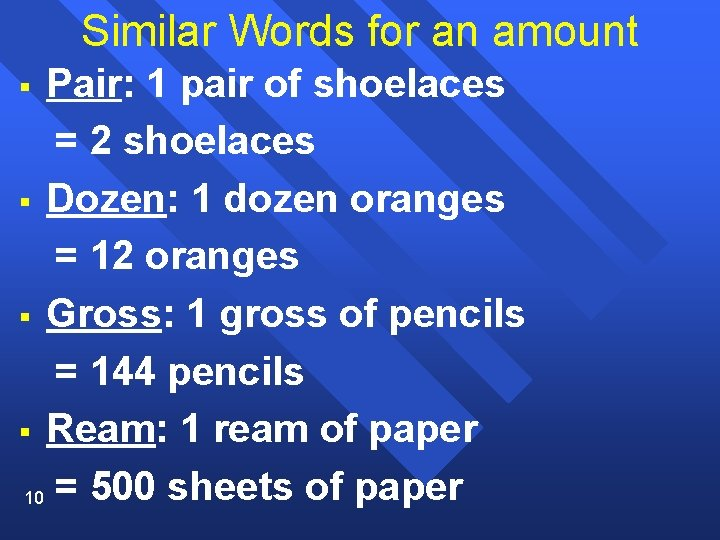Similar Words for an amount Pair: 1 pair of shoelaces = 2 shoelaces § Dozen: 1 dozen oranges = 12 oranges § Gross: 1 gross of pencils = 144 pencils § Ream: 1 ream of paper 10 = 500 sheets of paper §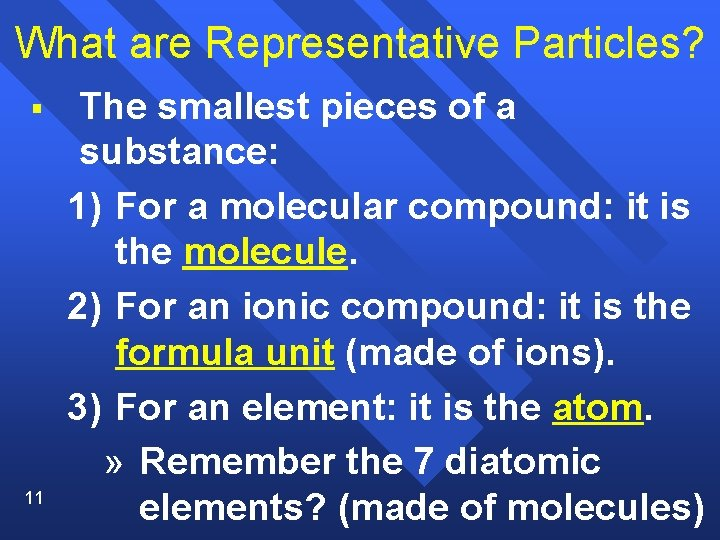What are Representative Particles? § 11 The smallest pieces of a substance: 1) For a molecular compound: it is the molecule. 2) For an ionic compound: it is the formula unit (made of ions). 3) For an element: it is the atom. » Remember the 7 diatomic elements? (made of molecules)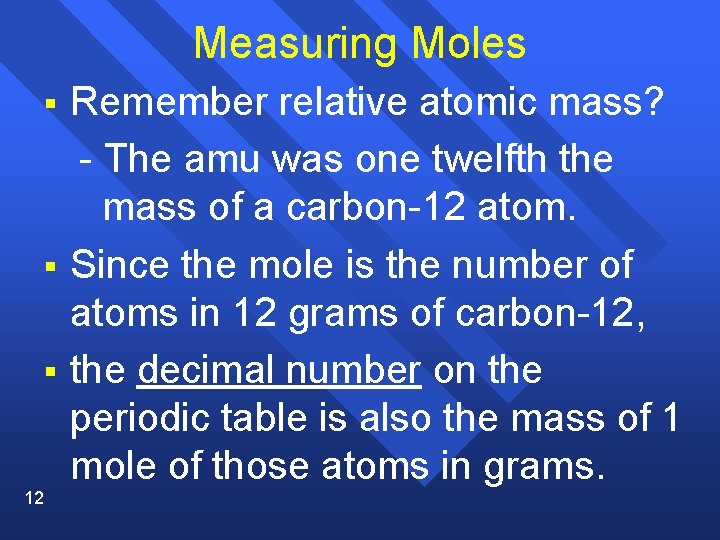Measuring Moles § § § 12 Remember relative atomic mass? - The amu was one twelfth the mass of a carbon-12 atom. Since the mole is the number of atoms in 12 grams of carbon-12, the decimal number on the periodic table is also the mass of 1 mole of those atoms in grams.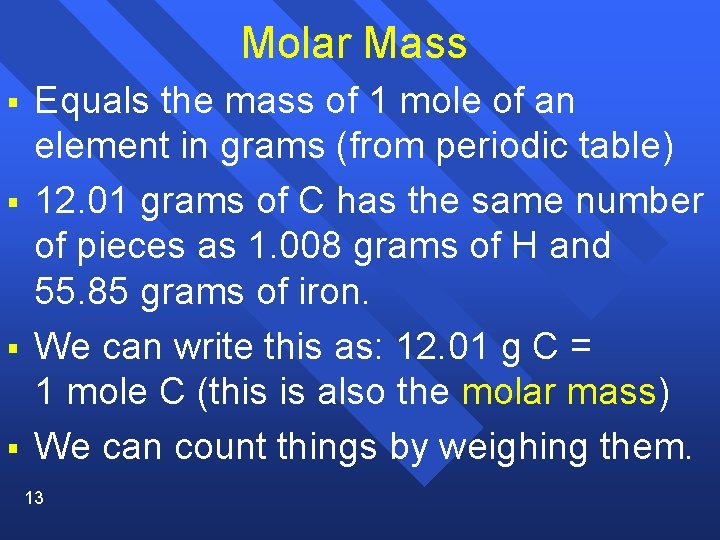Molar Mass § § Equals the mass of 1 mole of an element in grams (from periodic table) 12. 01 grams of C has the same number of pieces as 1. 008 grams of H and 55. 85 grams of iron. We can write this as: 12. 01 g C = 1 mole C (this is also the molar mass) We can count things by weighing them. 13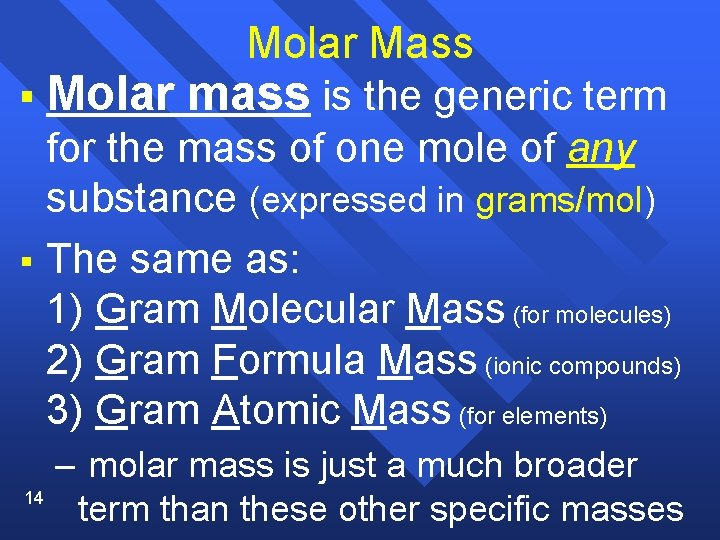Molar Mass § Molar mass is the generic term for the mass of one mole of any substance (expressed in grams/mol) § The same as: 1) Gram Molecular Mass (for molecules) 2) Gram Formula Mass (ionic compounds) 3) Gram Atomic Mass (for elements) 14 – molar mass is just a much broader term than these other specific massesWhat about compounds? § § 15 in 1 mole of H 2 O molecules there are two moles of H atoms and 1 mole of O atoms (think of a compound as a molar ratio) To find the mass of one mole of a compound –determine the number of moles of the elements present –Multiply the number times their mass (from the periodic table) –add them up for the total massCalculating Formula Mass Calculate the formula mass of magnesium carbonate, Mg. CO 3. 24. 3 g 16 + 12 g + 3 x (16. 00 g) = 84. 3 g Thus, 84. 3 grams is the formula mass for Mg. CO 3.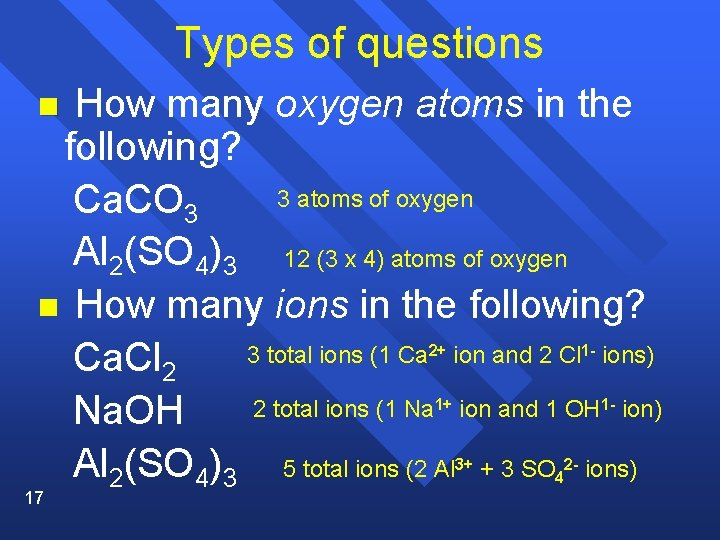Types of questions How many oxygen atoms in the following? 3 atoms of oxygen Ca. CO 3 Al 2(SO 4)3 12 (3 x 4) atoms of oxygen n How many ions in the following? 3 total ions (1 Ca ion and 2 Cl ions) Ca. Cl 2 2 total ions (1 Na ion and 1 OH ion) Na. OH Al 2(SO 4)3 5 total ions (2 Al + 3 SO ions) n 2+ 1 - 1+ 1 - 3+ 17 4 2 -Examples Calculate the molar mass of the following and tell what type it is: = 78 g/mol gram formula mass Na 2 S = 92 g/mol gram molecular mass N 2 O 4 C = 12 g/mol gram atomic mass Ca(NO 3)2 = 164 g/mol gram formula mass C 6 H 12 O 6 = 180 g/mol gram molecular mass (NH 4)3 PO 4 = 149 g/mol gram formula mass § 18For example § How many moles is 5. 69 g of Na. OH? (Solution on next slides) 19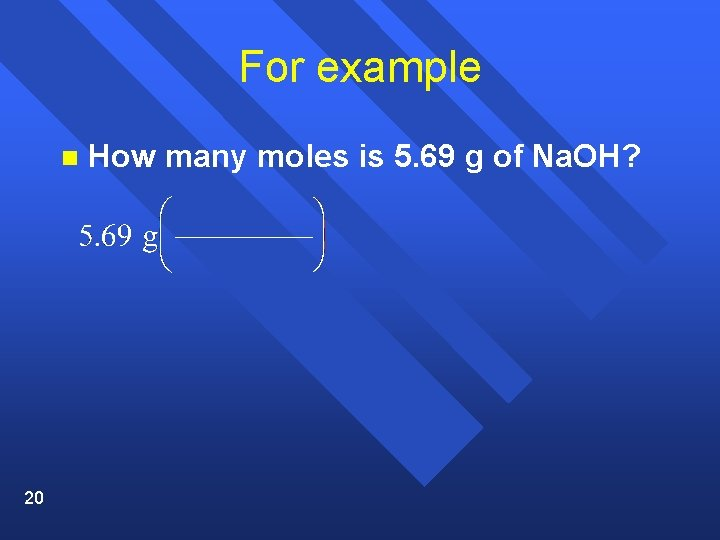For example n 20 How many moles is 5. 69 g of Na. OH?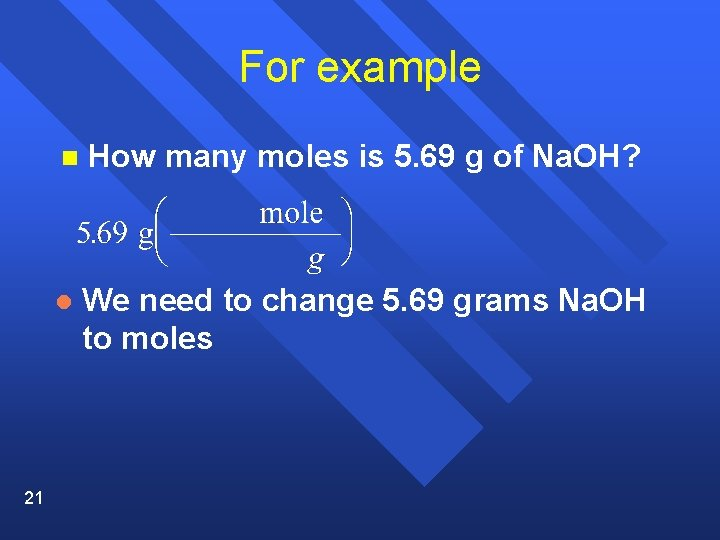For example 21 n How many moles is 5. 69 g of Na. OH? l We need to change 5. 69 grams Na. OH to moles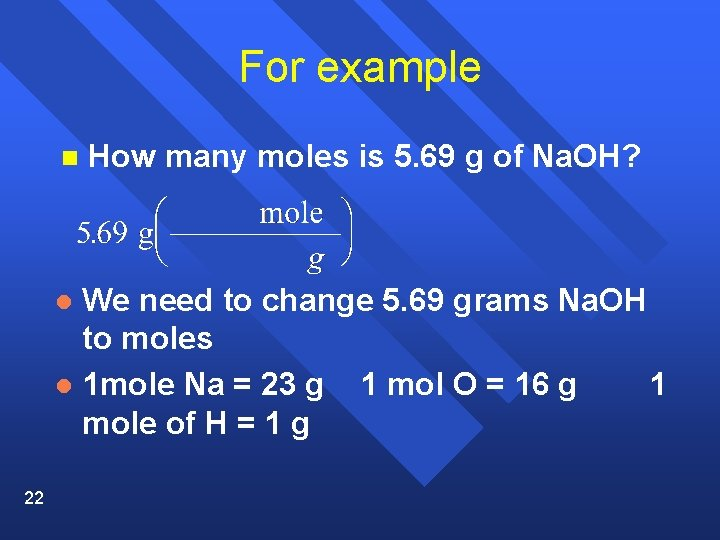For example n How many moles is 5. 69 g of Na. OH? We need to change 5. 69 grams Na. OH to moles l 1 mole Na = 23 g 1 mol O = 16 g 1 mole of H = 1 g l 22For example n How many moles is 5. 69 g of Na. OH? We need to change 5. 69 grams Na. OH to moles l 1 mole Na = 23 g 1 mol O = 16 g 1 mole of H = 1 g l 1 mole Na. OH = 40 g l 23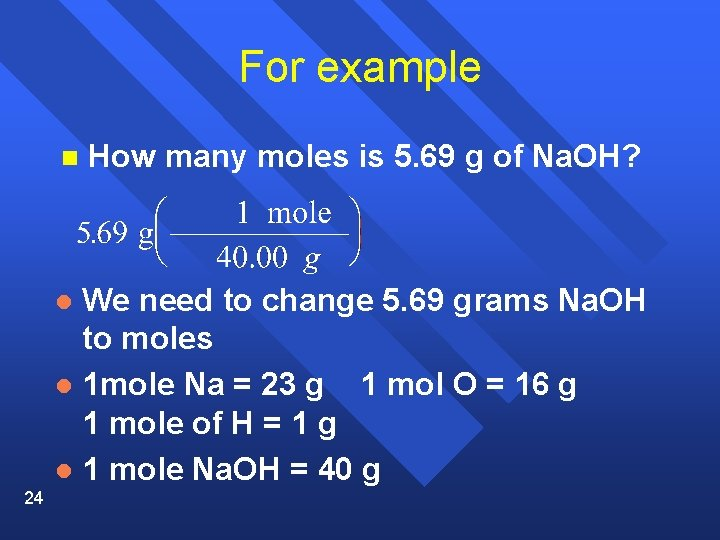For example n How many moles is 5. 69 g of Na. OH? We need to change 5. 69 grams Na. OH to moles l 1 mole Na = 23 g 1 mol O = 16 g 1 mole of H = 1 g l 1 mole Na. OH = 40 g l 24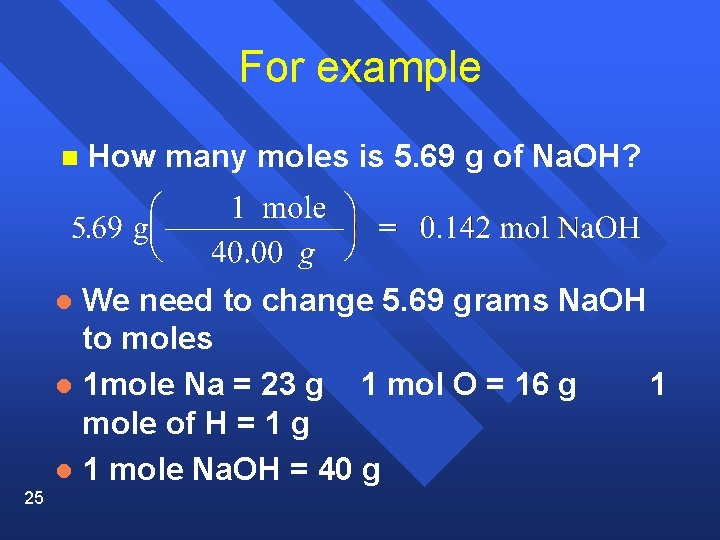For example n How many moles is 5. 69 g of Na. OH? We need to change 5. 69 grams Na. OH to moles l 1 mole Na = 23 g 1 mol O = 16 g 1 mole of H = 1 g l 1 mole Na. OH = 40 g l 25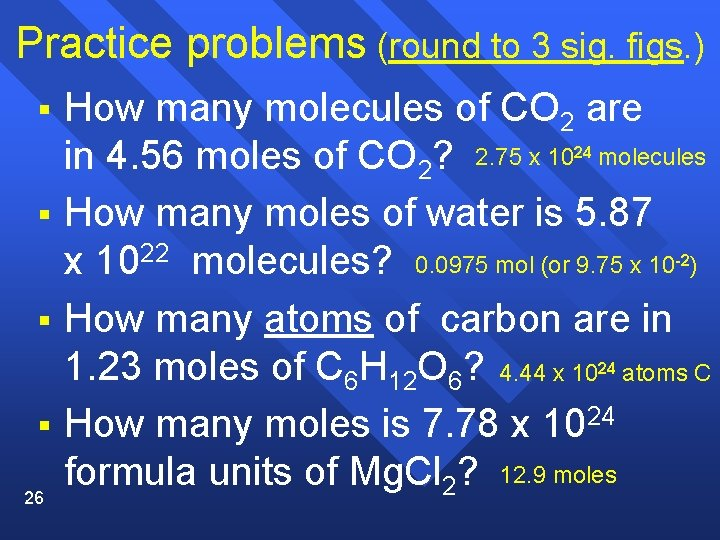Practice problems (round to 3 sig. figs. ) § How many molecules of CO 2 are in 4. 56 moles of CO 2? 2. 75 x 10 molecules How many moles of water is 5. 87 x 1022 molecules? 0. 0975 mol (or 9. 75 x 10 ) How many atoms of carbon are in 1. 23 moles of C 6 H 12 O 6? 4. 44 x 10 atoms C How many moles is 7. 78 x 1024 formula units of Mg. Cl 2? 12. 9 moles 24 § -2 § 24 § 26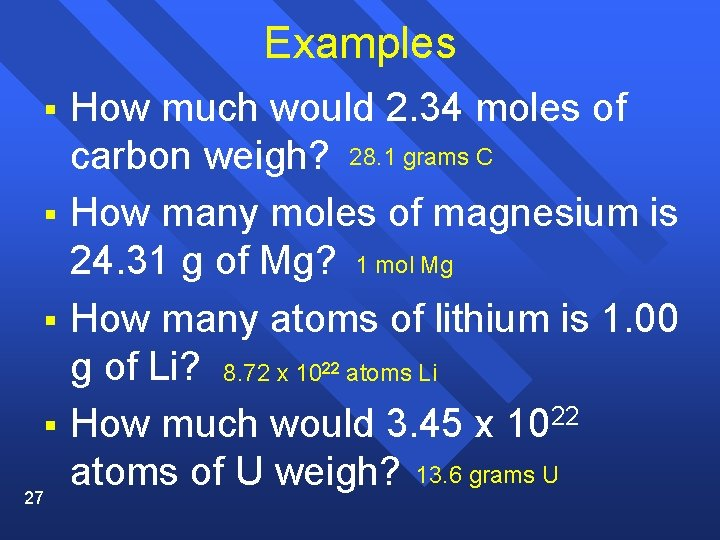Examples § § § How much would 2. 34 moles of carbon weigh? 28. 1 grams C How many moles of magnesium is 24. 31 g of Mg? 1 mol Mg How many atoms of lithium is 1. 00 g of Li? 8. 72 x 10 atoms Li How much would 3. 45 x 1022 atoms of U weigh? 13. 6 grams U 22 § 27Section 10. 2 Mole-Mass and Mole-Volume Relationships n OBJECTIVES: 28 –Describe how to convert the mass of a substance to the number of moles of a substance, and moles to mass.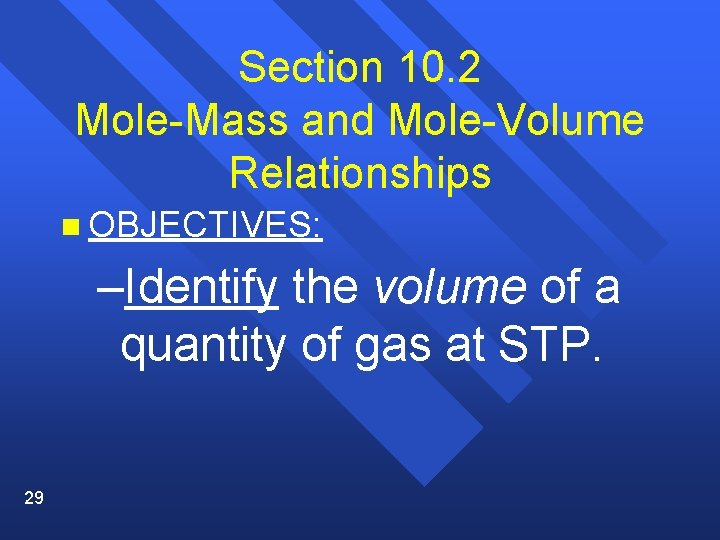Section 10. 2 Mole-Mass and Mole-Volume Relationships n OBJECTIVES: –Identify the volume of a quantity of gas at STP. 29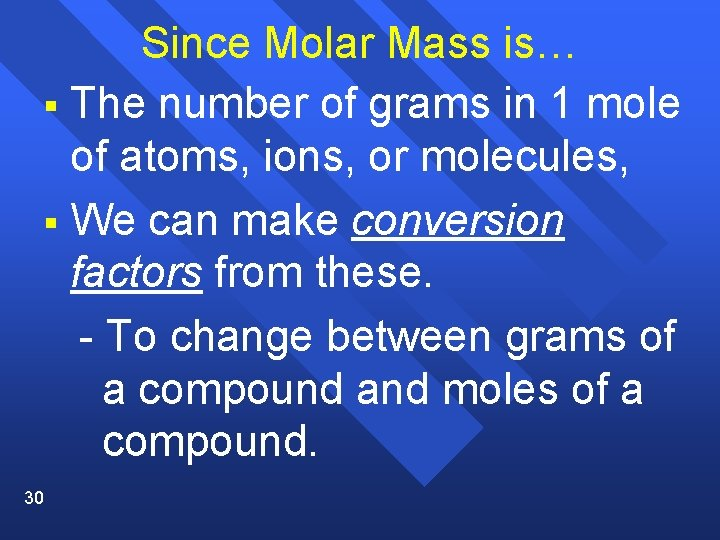Since Molar Mass is… § The number of grams in 1 mole of atoms, ions, or molecules, § We can make conversion factors from these. - To change between grams of a compound and moles of a compound. 30The Mole-Volume Relationship § Many of the chemicals we deal with are in the physical state as: gases. - They are difficult to weigh (or mass). § But, we may still need to know how many moles of gas we have. § Two things effect the volume of a gas: a) Temperature and b) Pressure § We need to compare all gases at the 31 same temperature and pressure.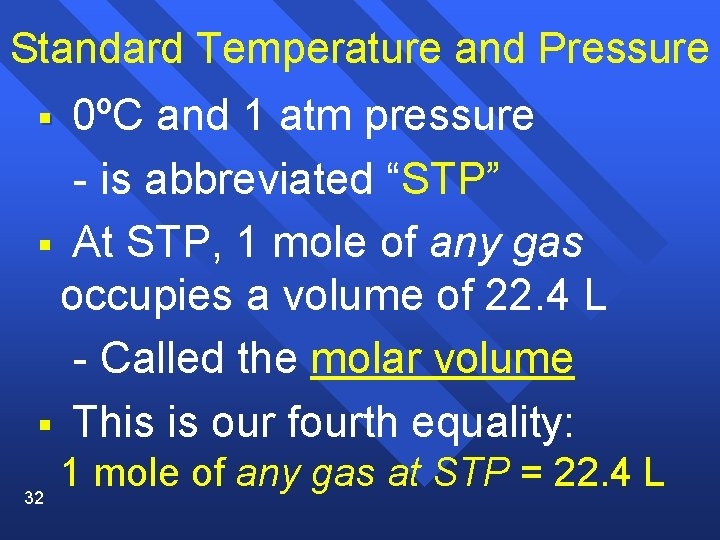Standard Temperature and Pressure 0ºC and 1 atm pressure - is abbreviated “STP” § At STP, 1 mole of any gas occupies a volume of 22. 4 L - Called the molar volume § This is our fourth equality: § 32 1 mole of any gas at STP = 22. 4 L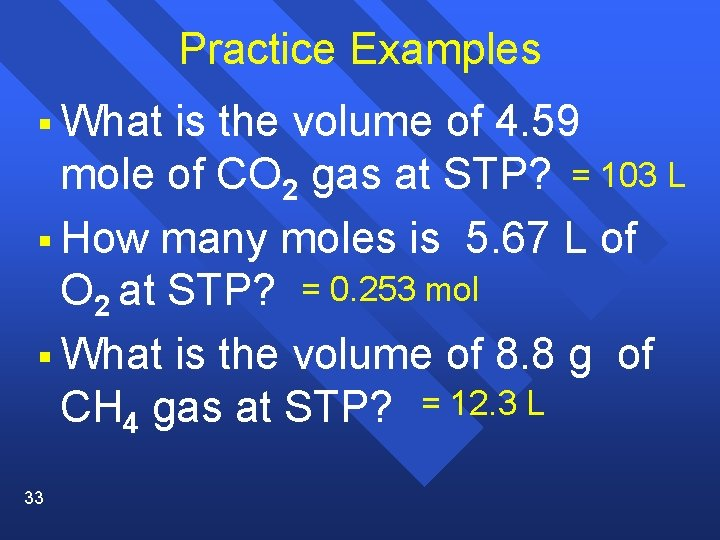Practice Examples § What is the volume of 4. 59 mole of CO 2 gas at STP? = 103 L § How many moles is 5. 67 L of O 2 at STP? = 0. 253 mol § What is the volume of 8. 8 g of CH 4 gas at STP? = 12. 3 L 33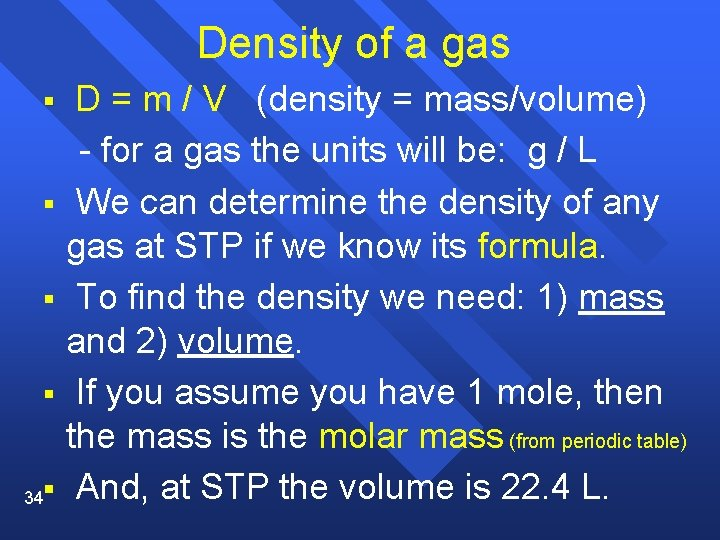Density of a gas D = m / V (density = mass/volume) - for a gas the units will be: g / L § We can determine the density of any gas at STP if we know its formula. § To find the density we need: 1) mass and 2) volume. § If you assume you have 1 mole, then the mass is the molar mass (from periodic table) 34§ And, at STP the volume is 22. 4 L. §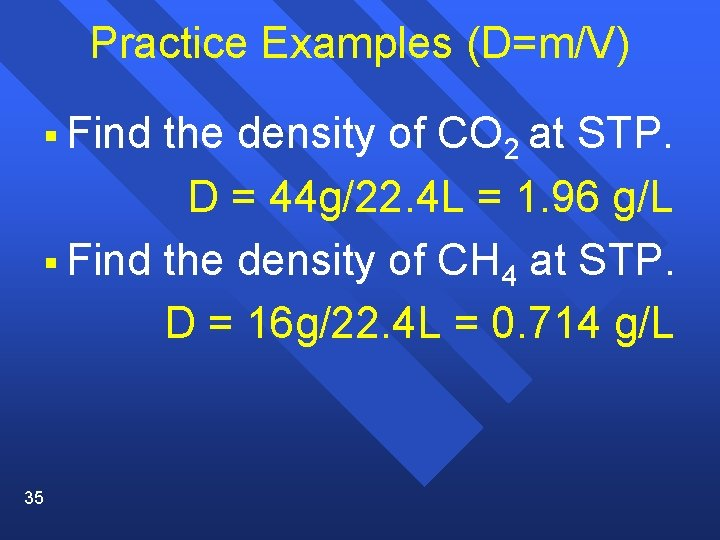Practice Examples (D=m/V) § Find the density of CO 2 at STP. D = 44 g/22. 4 L = 1. 96 g/L § Find the density of CH 4 at STP. D = 16 g/22. 4 L = 0. 714 g/L 35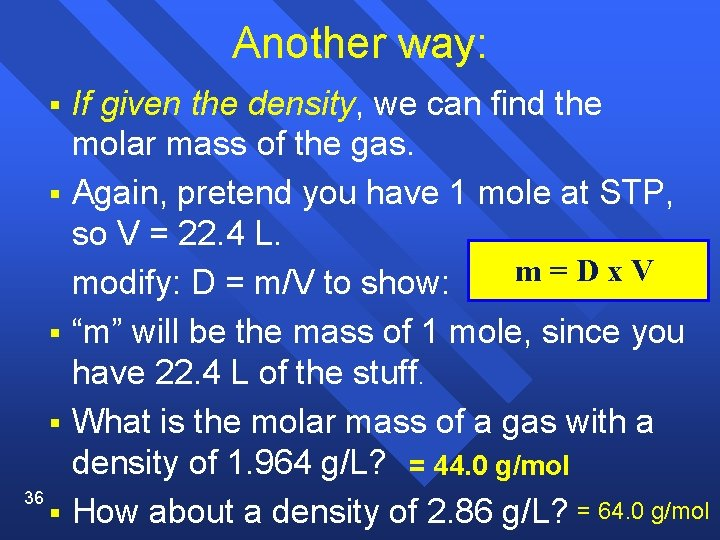Another way: § § 36 § If given the density, we can find the molar mass of the gas. Again, pretend you have 1 mole at STP, so V = 22. 4 L. m=Dx. V modify: D = m/V to show: “m” will be the mass of 1 mole, since you have 22. 4 L of the stuff. What is the molar mass of a gas with a density of 1. 964 g/L? = 44. 0 g/mol How about a density of 2. 86 g/L? = 64. 0 g/molSummary n These four items are all equal: a) 1 mole b) molar mass (in grams/mol) c) 6. 02 x 1023 representative particles (atoms, molecules, or formula units) d) 22. 4 L of gas at STP Thus, we can make conversion factors from these 4 values! 37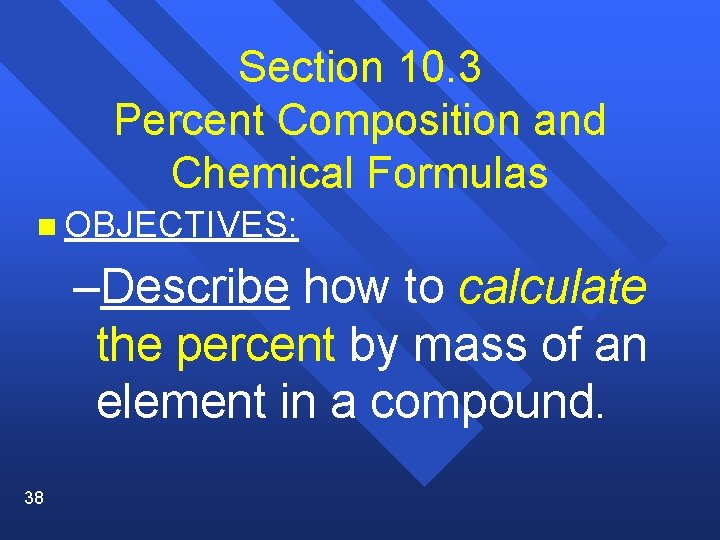Section 10. 3 Percent Composition and Chemical Formulas n OBJECTIVES: –Describe how to calculate the percent by mass of an element in a compound. 38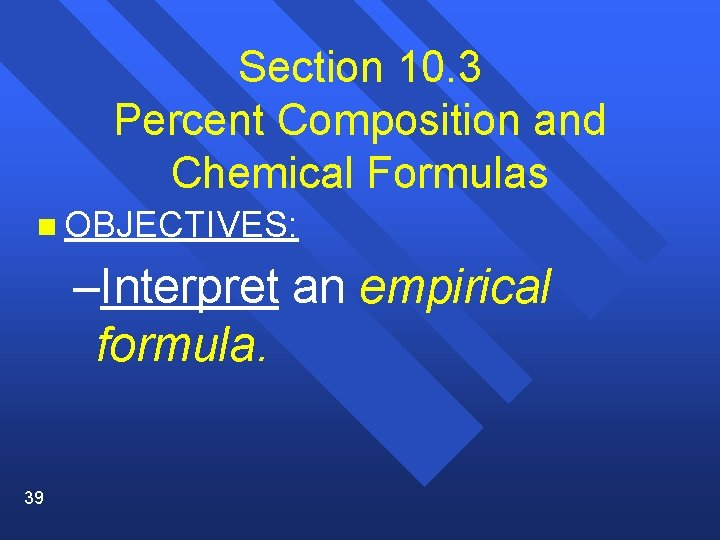Section 10. 3 Percent Composition and Chemical Formulas n OBJECTIVES: –Interpret an empirical formula. 39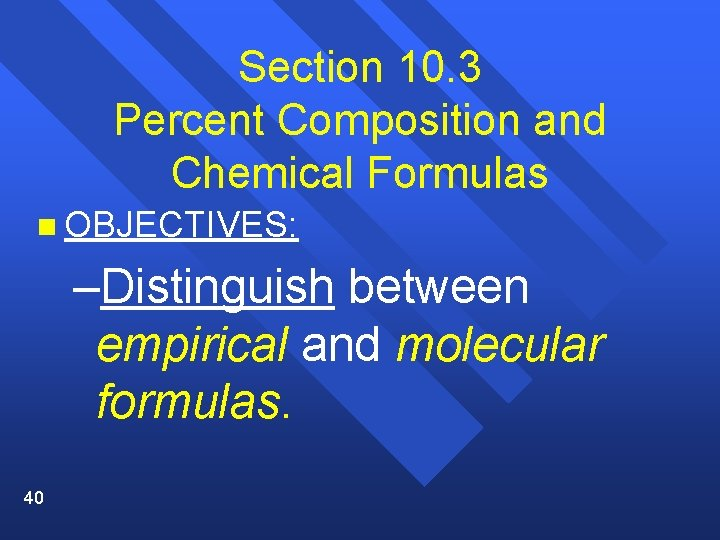Section 10. 3 Percent Composition and Chemical Formulas n OBJECTIVES: –Distinguish between empirical and molecular formulas. 40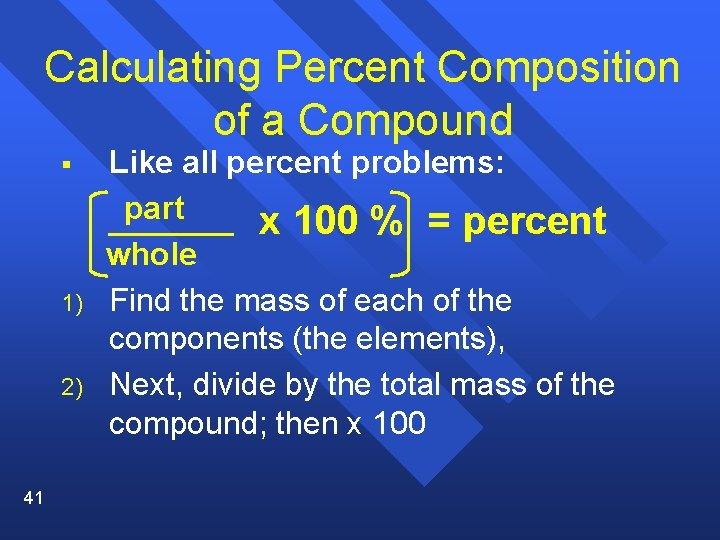Calculating Percent Composition of a Compound § 1) 2) 41 Like all percent problems: part x 100 % = percent whole Find the mass of each of the components (the elements), Next, divide by the total mass of the compound; then x 100Example § Calculate the percent composition of a compound that is made of 29. 0 grams of Ag with 4. 30 grams of S. 29. 0 g Ag X 100 = 87. 1 % Ag 33. 3 g total 4. 30 g S X 100 = 12. 9 % S 33. 3 g total 42 Total = 100 %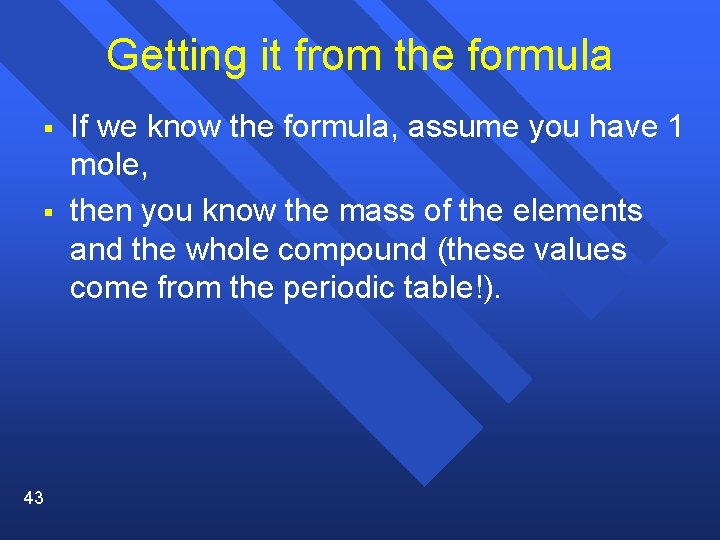Getting it from the formula § § 43 If we know the formula, assume you have 1 mole, then you know the mass of the elements and the whole compound (these values come from the periodic table!).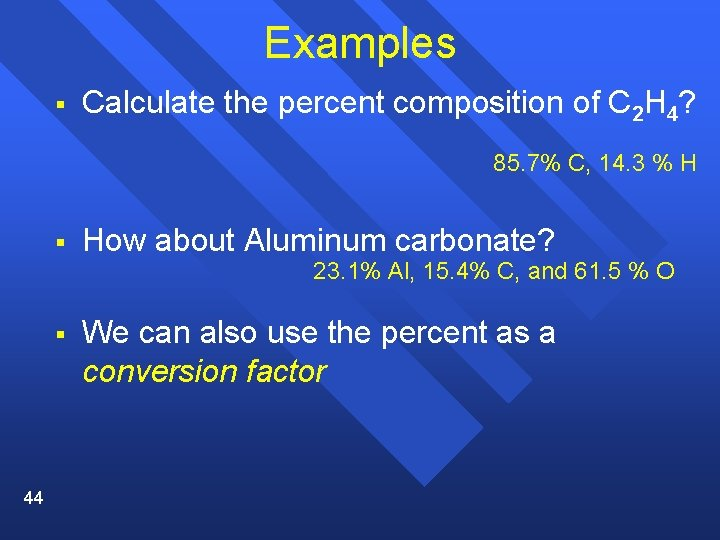Examples § Calculate the percent composition of C 2 H 4? 85. 7% C, 14. 3 % H § How about Aluminum carbonate? 23. 1% Al, 15. 4% C, and 61. 5 % O § 44 We can also use the percent as a conversion factor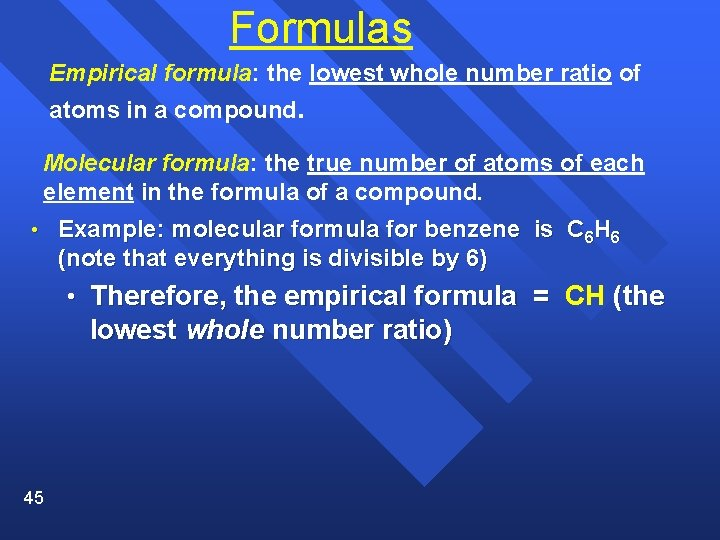Formulas Empirical formula: the lowest whole number ratio of atoms in a compound. Molecular formula: the true number of atoms of each element in the formula of a compound. • Example: molecular formula for benzene is C 6 H 6 (note that everything is divisible by 6) • Therefore, the empirical formula = CH (the lowest whole number ratio) 45Formulas (continued) Formulas for ionic compounds are ALWAYS empirical (the lowest whole number ratio = cannot be reduced). Examples: Na. Cl 46 Mg. Cl 2 Al 2(SO 4)3 K 2 CO 3Formulas (continued) Formulas for molecular compounds MIGHT be empirical (lowest whole number ratio). Molecular: (Correct formula) Empirical: (Lowest whole number ratio) 47 H 2 O C 6 H 12 O 6 C 12 H 22 O 11 H 2 O CH 2 O C 12 H 22 O 11Calculating Empirical § § 48 Just find the lowest whole number ratio C 6 H 12 O 6 = CH 2 O CH 4 N = this is already the lowest ratio. A formula is not just the ratio of atoms, it is also the ratio of moles. In 1 mole of CO 2 there is 1 mole of carbon and 2 moles of oxygen. In one molecule of CO 2 there is 1 atom of C and 2 atoms of O.Calculating Empirical § 1) We can get a ratio from the percent composition. Assume you have a 100 g sample - the percentage become grams (75. 1% = 75. 1 grams) 2) 3) 49 Convert grams to moles. Find lowest whole number ratio by dividing each number of moles by the smallest value.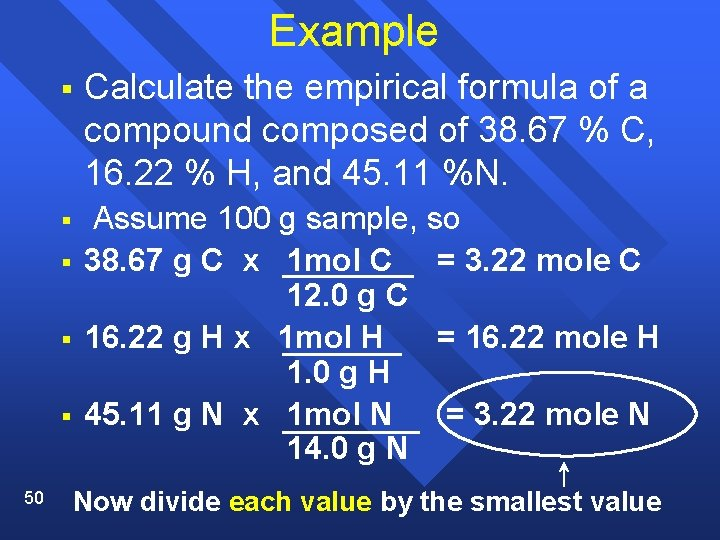Example § Calculate the empirical formula of a compound composed of 38. 67 % C, 16. 22 % H, and 45. 11 %N. § Assume 100 g sample, so 38. 67 g C x 1 mol C = 3. 22 mole C 12. 0 g C 16. 22 g H x 1 mol H = 16. 22 mole H 1. 0 g H 45. 11 g N x 1 mol N = 3. 22 mole N 14. 0 g N § § § 50 Now divide each value by the smallest value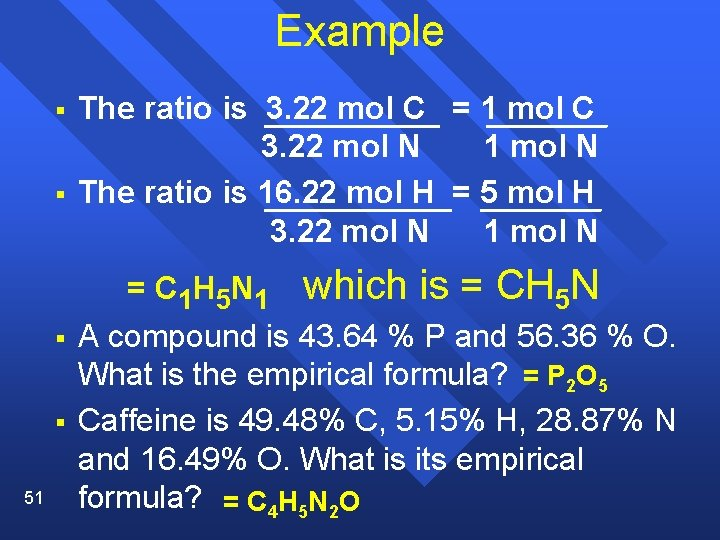Example § § The ratio is 3. 22 mol C = 1 mol C 3. 22 mol N 1 mol N The ratio is 16. 22 mol H = 5 mol H 3. 22 mol N 1 mol N = C 1 H 5 N 1 § § 51 which is = CH 5 N A compound is 43. 64 % P and 56. 36 % O. What is the empirical formula? = P 2 O 5 Caffeine is 49. 48% C, 5. 15% H, 28. 87% N and 16. 49% O. What is its empirical formula? = C 4 H 5 N 2 OEmpirical to molecular Since the empirical formula is the lowest ratio, the actual molecule would weigh more. §By a whole number multiple. § Divide the actual molar mass by the empirical formula mass – you get a whole number to increase each coefficient in the empirical formula § Caffeine has a molar mass of 194 g. 52 what is its molecular formula? = C 8 H 10 N 4 O 2 §Note page 313 – Gas Chromatography used for chemical analysis 5354